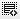## What is the yearly effective rate of interest?0
A bank charges 8.5% p.a. interest on loan on a monthly reducing balance basis. What is the yearly effective rate of interest?

How should we calculate?0

((1 + (annualized interest rate/frequency))^ frequency - 1) x100

For the above question -
((1+(0.085/12))^12-1)*100 = 8.9%0

Hi. You can do the above calculation with in-built function "EFFECT" in Excel. Put the Nominal rate = 8.5% and Npery = 12 as one year has 12 months i.e. 12 times compounding in one year.
However, remembering the formula is a nice thing to do.

Click on thisicon to add code snippet.